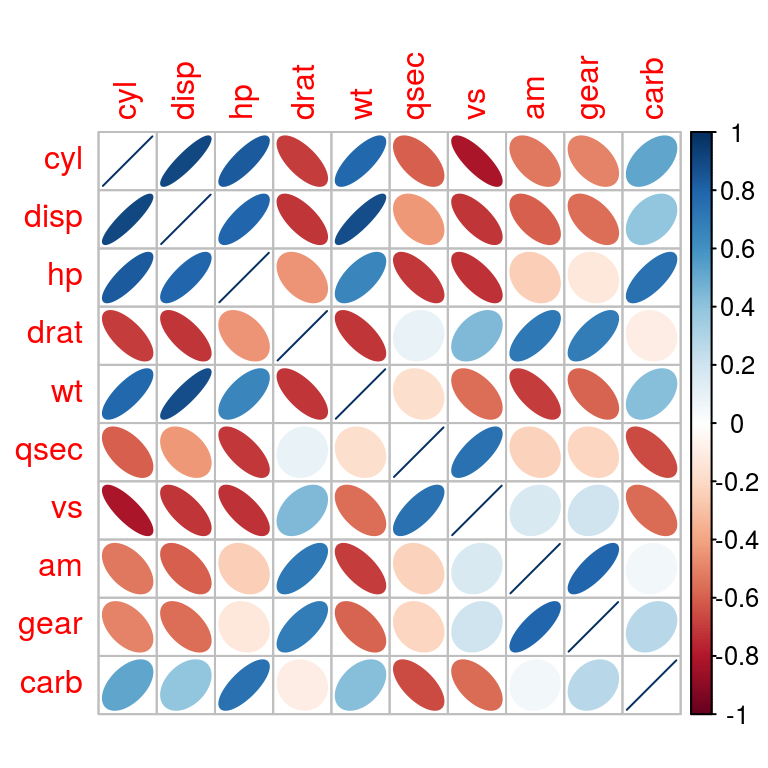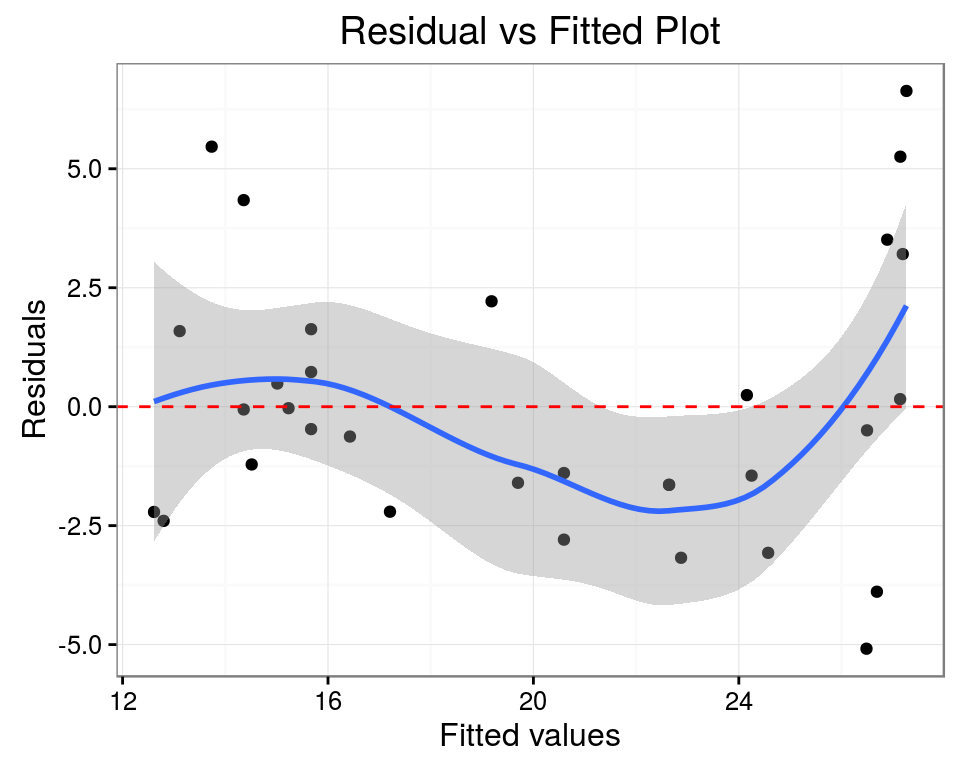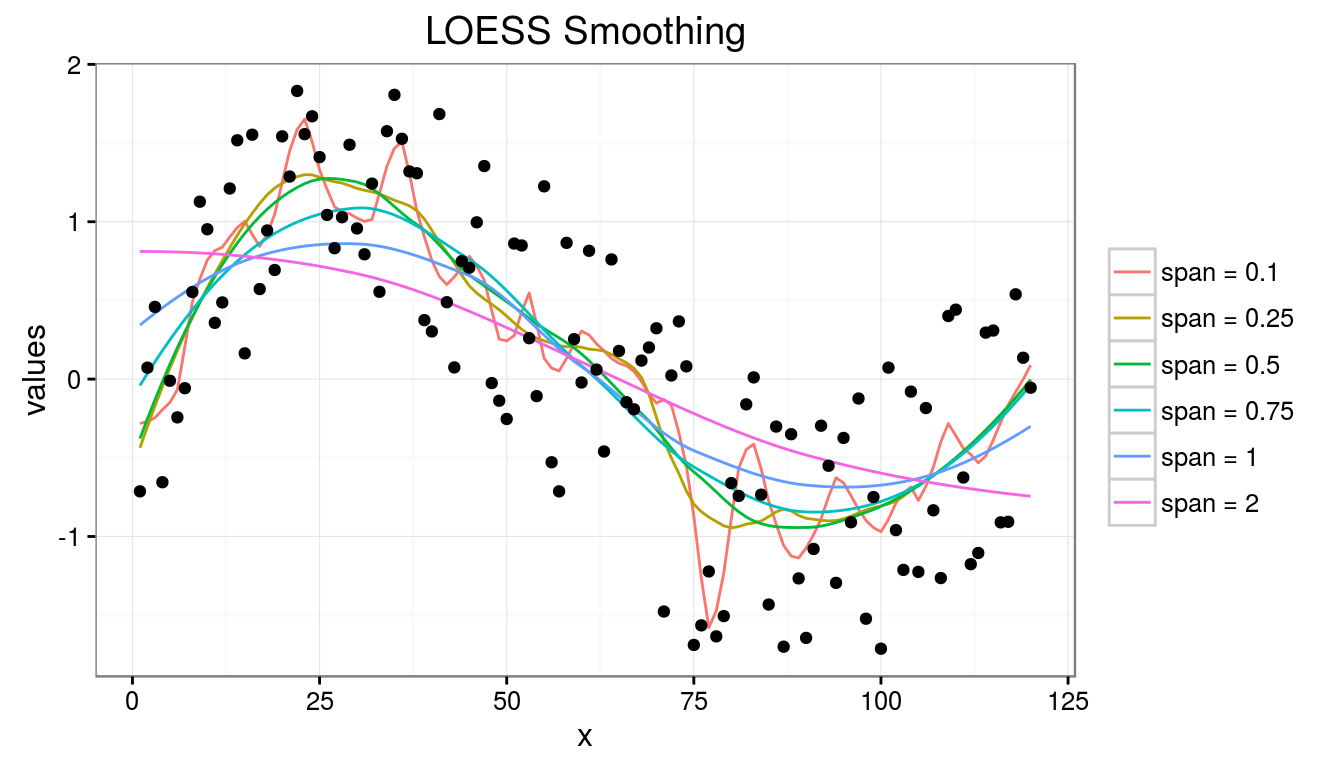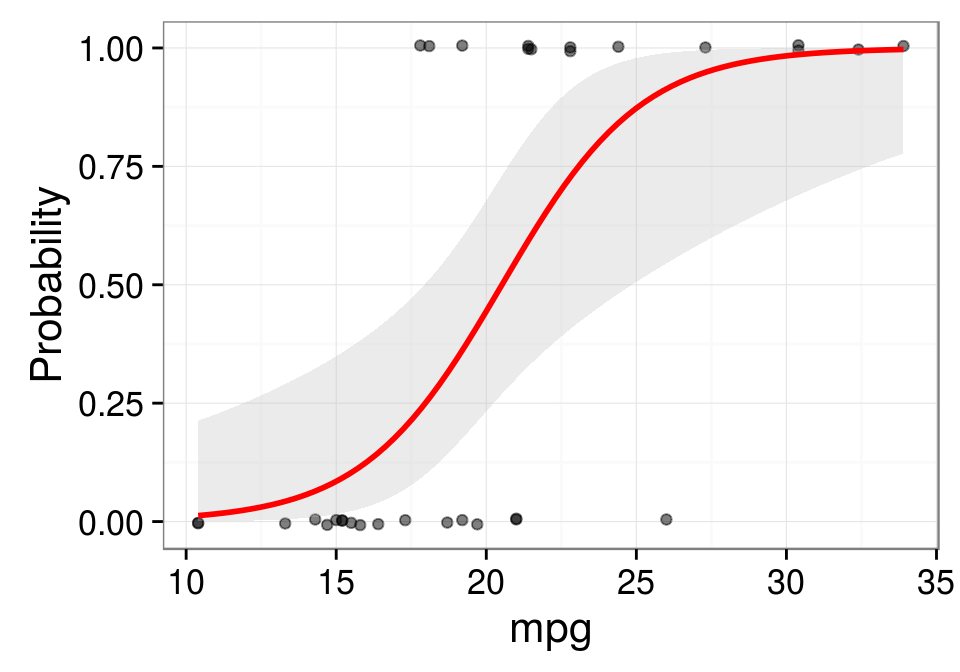## Dataset

A data frame with 32 observations on 11 variables.

var Description
mpg Miles/(US) gallon
cyl Number of cylinders
disp Displacement (cu.in.)
hp Gross horsepower
drat Rear axle ratio
wt Weight (1000 lbs)
qsec 1/4 mile time
vs V/S
am Transmission (0 = automatic, 1 = manual)
gear Number of forward gears
carb Number of carburetors
head(mtcars)
##                    mpg cyl disp  hp drat    wt  qsec vs am gear carb
## Mazda RX4         21.0   6  160 110 3.90 2.620 16.46  0  1    4    4
## Mazda RX4 Wag     21.0   6  160 110 3.90 2.875 17.02  0  1    4    4
## Datsun 710        22.8   4  108  93 3.85 2.320 18.61  1  1    4    1
## Hornet 4 Drive    21.4   6  258 110 3.08 3.215 19.44  1  0    3    1
## Hornet Sportabout 18.7   8  360 175 3.15 3.440 17.02  0  0    3    2
## Valiant           18.1   6  225 105 2.76 3.460 20.22  1  0    3    1## OLS Regression

Given a data set $${\{y_{i},\,x_{i1},\ldots ,x_{ip}\}_{i=1}^{n}}$$ of n statistical units, the model takes the form: $y_{i}=\beta _{1}x_{i1}+\cdots +\beta _{p}x_{ip}+\varepsilon _{i}=\mathbf {x} _{i}^{\rm {T}}{\boldsymbol {\beta }}+\varepsilon _{i},\qquad i=1,\ldots ,n,$ where,
$$y_{i}$$ is the response variable,
$$x_{i1},\,x_{i2},\,\ldots ,\,x_{ip}$$ are the regressors,
$$\beta$$ is a p-dimensional parameter vector (estimated by least-squares method)
$$\epsilon_{i}$$ is the error term.

### Fitting the Model

We will regress the mpg variable onto disp, cyl, am. A linear model is fitted by least-squares, which minimizes the sum of squared residuals and leads to a closed-form expression for the estimated value of the unknown parameter $$\boldsymbol \beta$$.

Objective: ${\hat {\boldsymbol {\beta }}}={\rm {arg}}\min _{\boldsymbol \beta }\,\left\lVert \mathbf {y}-\mathbf {X}{\boldsymbol \beta } \right\rVert ,$

which leads to: ${\hat {\boldsymbol {\beta }}}=(\mathbf {X} ^{\rm {T}}\mathbf {X} )^{-1}\mathbf {X} ^{\rm {T}}\mathbf {y} ={\big (}\,{\textstyle \sum }\mathbf {x} _{i}\mathbf {x} _{i}^{\rm {T}}\,{\big )}^{-1}{\big (}\,{\textstyle \sum }\mathbf {x} _{i}y_{i}\,{\big )}.$

model <- lm(mpg ~ cyl + disp + am, data = mtcars)
model$coefficients ## (Intercept) cyl disp am ## 32.91685995 -1.61821911 -0.01559041 1.92872540 Adjusted R-squared: 0.7521509 F-statistic: 32.3586942# sales vs predicted sales head(data.frame(mpg = mtcars$mpg, pred = predict(model)), 10)
##                    mpg     pred
## Mazda RX4         21.0 22.64180
## Mazda RX4 Wag     21.0 22.64180
## Datsun 710        22.8 26.68894
## Hornet 4 Drive    21.4 19.18522
## Hornet Sportabout 18.7 14.35856
## Valiant           18.1 19.69970
## Duster 360        14.3 14.35856
## Merc 240D         24.4 24.15687
## Merc 230          22.8 24.24885
## Merc 280          19.2 20.59459

## LOESS

Suppose a dataset consists of $$N$$ pairs of observations, $$\{(x_i, y_i)\}_{i=1}^N$$. For fitting a point $$x$$, define a bandwidth $$h(x)$$ and a smoothing window $$(x−h(x),\,x+h(x))$$. The LOWESS algorithm finds the vector $$\boldsymbol\beta$$ of coefficients which minimizes the locally weighted sum of squares $\boldsymbol{\hat\beta} = \underset {\boldsymbol\beta \in \mathbb R^{p + 1}}{\arg\min} \sum_{i=1}^N w_i(x)(y_i - \langle{\boldsymbol\beta, \, A(x_i - x)}\rangle)^2$ where,
\begin{align} A_0(v) = 1, && A_k(v) = \frac {v^k}{k!}, && w_i(x) = W\left(\frac {x_i - x}{h(x)}\right), \end{align} $$W(u)$$ is a weight function that assigns largest weight to observations close to $$x$$.

The local regression estimate is $\hat f(x) = \langle \boldsymbol {\hat\beta}, \, A(0) \rangle = \boldsymbol {\hat\beta}_0.$

set.seed(19)

period <- 120
x <- 1:period
y <- sin(2*pi*x/period) + runif(length(x),-1,1)

spanlist <- c(0.10, 0.25, 0.50, 0.75, 1.00, 2.00)
loess.lines <- sapply(spanlist, function(s) {
y.loess <- loess(y ~ x, span = s, data.frame(x = x, y = y))
cat("span = ", format(s, nsmall = 2), ", rse: ", y.loess\$s, "\n", sep = "")
predict(y.loess)
})
## span = 0.10, rse: 0.5825607
## span = 0.25, rse: 0.5787946
## span = 0.50, rse: 0.5719129
## span = 0.75, rse: 0.5816912
## span = 1.00, rse: 0.6184237
## span = 2.00, rse: 0.7045324## Logistic Regression

Formally, the logistic regression model is that $\log {\frac {p(x)}{1 - p(x)}} = \beta_0 + \beta^T x.$ Suppose that we are given a sample $$(x_i, y_i)$$, $$i = 1,\ldots,n$$, where $$y_i$$ denotes the class $$\in \{1, 2\}$$ of the $$i$$-th observation. Then, assuming that the class labels are conditionally independent, the log-likelihood is given by \begin{aligned} \ell(\beta_0, \beta) &= \sum_{i=1}^n \log {P(C = y_i \mid X = x_i)} &\\ &= \sum_{i=1}^n \left\{u_i \cdot (\beta_0 + \beta^T x_i) - \log {(1 + \exp (\beta_0 + \beta^T x_i))} \right\} \end{aligned} where, \begin{align} u_i = \begin{cases} 1 & {\mbox{if }} y_i = 1 \\ 0 & {\mbox{if }} y_i = 2 \end{cases} \end{align}

The coefficients are estimated by maximizing the likelihood, $\hat\beta_0, \hat\beta = \underset {\beta_0 \in \mathbb R, \beta \in \mathbb R^p}{\arg \max} \ell(\beta_0, \beta)$

mtcars.glm <- glm(vs ~ mpg, data = mtcars, family = binomial)
mtcars.glm
##
## Call:  glm(formula = vs ~ mpg, family = binomial, data = mtcars)
##
## Coefficients:
## (Intercept)          mpg
##     -8.8331       0.4304
##
## Degrees of Freedom: 31 Total (i.e. Null);  30 Residual
## Null Deviance:       43.86
## Residual Deviance: 25.53     AIC: 29.53## Remarks

In linear regression, the intercept coefficient is the expected value of the response when the predictor variables is zero, and the slopes represent the expected change in response for a unit change in the corresponding predictor. In the linear regression example, the average value of value of mpg is 32.9168599 and for every 1 unit increase in cyl, mpg changes by -1.6182191 units. Similarly, for every 1 unit increase in disp, mpg changes by -0.0155904 units. Regression is an enormously important tool for business and financial analysis. As another example, we may choose the rate of inflation as the target variable, and create a regression model to estimate it with the following variables: GDP, import-to-export ratio, number of unemployed as the predictors. A crude NASDAQ stock trading could be modeled as: $(\% {Number \, of \, Trades}_t^{\lambda} - 1) \, / \, \lambda = \beta_0 + \beta_1 {Firm Size}_t + \beta_2 {Volatility}_t + \beta_3 {TradeSize}_t + \beta_4 {Price}_t + \epsilon_t$.

LOESS assumes no functional form and doesn’t produce a regression function or slope parameters that is easily represented by a mathematical formula.

The logistic slope can be interpreted as the effect of a unit change in predictor variable on the log odds ratio when the other variables in the model is held constant. The logistic intercept is the log odds estimate for the whole population. In the example for logistic regression, we can infer that the odds of having a V-engine, gets scaled by $$\mathrm{e}^{0.4304135} = 1.5378933$$ when mpg increases by one unit. Let us consider another example of Logistic Regression applied to medical domain. Lactate and Urea levels as metabolic markers can be used to investigate the survivability of a patient in accident and emergency unit. Suppose a logistic regression model could be constructed as follows to predict a patient’s death: $\log {\frac {p}{1 - p}} = -5.716 + (0.270 \times lactate) + (0.053 \times urea) + (1.425 \times age\_group)$. We can see that odds of death gets multiplied by $$\mathrm{e}^{0.270} = 1.31$$ for every one unit increase in lactate levels in serum.